Forum » Reverse Polish Notation!

## Reverse Polish Notation!Narvius 11 years ago http://en.wikipedia.org/wiki/Reverse_Polish_notationIn Ruby. Written reusably.Usage: Launch in command line, input RPN string. Enter.I less-than-three self-written, concise code.`class String def rpn # Reverse Polish Notation. stack, string = Array.new, self.strip while string[" "] midval, string = string[0, string.index(" ")], string[string.index(" "), string.length - string.index(" ")].strip (stack.push(eval("#{stack[-2]}#{midval}#{stack[-1]}")).push(stack.pop(3); next) unless ("0".."9") === midval stack.push(midval.to_i) end return eval("#{stack}#{string}#{stack}") endendputs gets.rpngets` # quoteE_net4 11 years ago Fun stuph. Let's make it in other languages.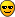I think I had to make something like that in some Programming class, but I don't mind searching. I'd rather rebuild it. # quoteNarvius 11 years ago I'm pretty sure MK will take care of a Python implementation xD # quoteAmarth 11 years ago Eh, I can barely understand that, sorry. :/ Especially the while condition and the syntax for eval are a bit unclear to me. Can you give some test cases, as in, input and output to your rpn function?Python code might be a bit longer. but won't be much longer. And it will *obviously* be more readable. I could think about a few others too I guess...And I'm still a bit wary towards the whole monkey-patching business... # quoteMageKing17 11 years ago I'd have to understand the algorithm to rewrite it in Python. # quoteNarvius 11 years ago I specifically wrote the Ruby one as short as possible. I could have made it more readable (and the first versions, in fact, were).Example input:1 2 + 4 * 5 6 7 - - -So, basically. You have a stack.Read the string from left to right.1 -> push onto stack (1)2 -> push onto stack (1 2)+ -> perform operation on two top items on stack, pop them, push result (3)4 -> push onto stack (3 4)* -> perform, pop, push (12)5 -> push (12 5)6 -> push (12 5 6)7 -> push (12 5 6 7)- -> perform, pop, push (12 5 -1)- -> perform, pop, push (12 6)- -> perform, pop, push (6)Output 6.Notes on the Ruby implementation:string[string2] returns string2 if string contains it, nil (ie. null, which obviously evaluates to false) otherwise. Therefore the while clause string[" "] makes the loop continue as long as the string contains a space. This is usually used with regexpes.midval stores the current token, string the unread part of the input.eval performs the string given as if it was actual code. #{expression} in a string evaluates the expression, calls to_s on the result and substitutes it for the #{}.Also, negative indices on arrays take items from the right. In for a = [1, 2, 3, 4, 5], a[-1] = 5."#{stack[-2]}#{midval}#{stack[-1]" could for example become "1+2", which then is evalled and returns 3 (which is pushed onto the stack).stack.push(eval("1+2")).push(stack.pop(3))push returns the stack itself, hence the chainpush.In my implementation, the stack (1 2) first becomes (1 2 3), and then (3), this is because some operations have a set order (substraction, for example), and I can't do #{stack.pop}#{midval}#{stack.pop}, because that'd reverse the order. So I just push the result, pop three items, repush the result.Once the string contains no more spaces, one last token is left in string. It has to be an operation, so I just perform it and return the result....that's pretty much everything I can think of. # quoteAmarth 11 years ago Pythonic solution:`def rpn(string): stack, tokens = [], string.strip().split(" ") for token in tokens: if not token.isdigit(): token = eval("%s%s%s" % (stack[-2],token,stack[-1]) stack = stack[:-2] stack.append(int(token)) return stackstring = raw_input("Your command? ")print rpn(string)`Some slight differences from your code, in particular I first split the string into tokens instead of parsing it in a while-loop, and I don't push on the stack for no reasons :p.Your code doesn't work for me btw:`rpn.rb:9:in `rpn': (eval):1:in `rpn': compile error (SyntaxError)(eval):1: syntax error, unexpected \$end from rpn.rb:13:in `eval' from rpn.rb:9:in `rpn' from rpn.rb:13`But I might be doing something wrong obviously.EDIT: Also, happy birthday! (commencing threadjack...) # quoteNarvius 11 years ago Thanks :>`class String def rpn # Reverse Polish Notation. stack, tokens = Array.new, self.strip.split(" ") for token in tokens (stack.push(eval("#{stack[-2]}#{token}#{stack[-1]}")).push(stack.pop(3); next) unless ("0".."9") === token stack.push(token.to_i) end return stack endendputs gets.rpngets`You're right, tokenizing it beforehand saves a line.And it works just fine for me. I used Ruby 1.9, btw. # quoteE_net4 11 years ago Here's an exhausting RPN Java class:`import java.util.ArrayDeque;import java.util.Deque;import java.util.Scanner;public class RPN { private Deque numberstack; public RPN() { numberstack = new ArrayDeque(); } public float run(String code) { Scanner sc = new Scanner(code); String t; float op1 = 0; float op2 = 0; short op = 0; while (sc.hasNext()) { t = sc.next(); op = getOperation(t); switch (op) { case 0: //Try to read value try { numberstack.push(Float.parseFloat(t)); } catch (java.lang.NumberFormatException exc) { //Nope, it's not a value. Simply ignore it. } break; default: //Operation. Get operands here. if (!numberstack.isEmpty()) { op2 = numberstack.pop(); if (!numberstack.isEmpty()) op1 = numberstack.pop(); else op1 = 0; } else op2 = 0; } if (op == 0) continue; switch (op) { case 1: //Add numberstack.push(op1 + op2); break; case 2: //Subtract numberstack.push(op1 - op2); break; case 3: //Multiply numberstack.push(op1 * op2); break; case 4: //Divide numberstack.push(op1 / op2); break; case 5: //Power { float acc = 1; for (int i = 0; i < op2 ; i++) { acc *= op1; } numberstack.push(acc); } break; } } sc.close(); return numberstack.size() != 0 ? numberstack.peekFirst() : 0; } private short getOperation(String s) { char c = s.charAt(0); //Operations: //0 - no valid operation. Is operand or nothing at all. //1 - add - + //2 - subtract - - //3 - multiply - * //4 - divide - / //5 - power - ^ switch (c) { case '+': return 1; case '-': return 2; case '*': return 3; case '/': return 4; case '^': return 5; } return 0; } }`It works with single-precision floating points, by the way. # quoteNarvius 11 years ago Oh, nice. The first "serious-looking" implementationYou might want to empty the stack at the end, so one instance can be reused - the stack is private, after all. # quoteE_net4 11 years ago What, like this?`import java.util.ArrayDeque;import java.util.Deque;import java.util.Scanner;public class RPN { private Deque numberstack; public RPN() { numberstack = new ArrayDeque(); } public float run(String code) { Scanner sc = new Scanner(code); String t; float op1 = 0; float op2 = 0; short op = 0; while (sc.hasNext()) { t = sc.next(); op = getOperation(t); switch (op) { case 0: //Try to read value try { numberstack.push(Float.parseFloat(t)); } catch (java.lang.NumberFormatException exc) { //Nope, it's not a value. Simply ignore it. } break; default: //Operation. Get operands here. if (!numberstack.isEmpty()) { op2 = numberstack.pop(); if (!numberstack.isEmpty()) op1 = numberstack.pop(); else op1 = 0; } else op2 = 0; } if (op == 0) continue; switch (op) { case 1: //Add numberstack.push(op1 + op2); break; case 2: //Subtract numberstack.push(op1 - op2); break; case 3: //Multiply numberstack.push(op1 * op2); break; case 4: //Divide numberstack.push(op1 / op2); break; case 5: //Power { float acc = 1; for (int i = 0; i < op2 ; i++) { acc *= op1; } numberstack.push(acc); } break; } } sc.close(); float res = numberstack.size() != 0 ? numberstack.peekFirst() : 0; while(!numberstack.isEmpty()) //there you go. :P numberstack.pop(); return res; } private short getOperation(String s) { char c = s.charAt(0); //Operations: //0 - no valid operation. Is operand or nothing at all. //1 - add - + //2 - subtract - - //3 - multiply - * //4 - divide - / //5 - power - ^ switch (c) { case '+': return 1; case '-': return 2; case '*': return 3; case '/': return 4; case '^': return 5; } return 0; } }`You sir, are correct.Also, I figured just now: I used both size() != 0 and !isEmpty() for the same thing.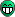# quoteAmarth 11 years ago Bah. That's way too procedural. While, break, continue, if-then-else... And you're using magic numbers. Also, why use Deque and not just Stack?Here is a fully bloated OOP Java way to fix the problem. It should be split among multiple files probably. Please note the complete lack of statics, while loops and if-then-elses (except in one position where I think it would be too much trouble to work around and another where it's basically some safety measure). Most of the work is actually in defining the structure around Tokens (basically a safer eval, which you get (probably unsafely) for free in Ruby and Python), once those are out it's not hard to get it working.`import java.util.ArrayList;import java.util.HashMap;import java.util.List;import java.util.Map;import java.util.Stack;public class RPN { private abstract class Operation { private String opString; public Operation(String opString) { this.opString = opString; } public String getOpString(){ return opString; } public abstract Integer doOp(Integer first, Integer second); } private class Addition extends Operation { public Addition() { super("+"); } public Integer doOp(Integer first, Integer second) { return first + second; } } private class Subtraction extends Operation { public Subtraction() { super("-"); } public Integer doOp(Integer first, Integer second) { return first - second; } } private class Multiplication extends Operation { public Multiplication() { super("*"); } public Integer doOp(Integer first, Integer second) { return first * second; } } private class Division extends Operation { public Division() { super("/"); } public Integer doOp(Integer first, Integer second) { return first / second; } } private class Token { private boolean isOperation; private Operation operation; private Integer number; public Token(Operation op){ isOperation = true; this.operation = op; } public Token(Integer number){ isOperation = false; this.number = number; } public boolean isOperation(){ return isOperation; } public Operation getOperation(){ if(!isOperation()){ throw new UnsupportedOperationException(); } return operation; } public Integer getInteger(){ if(isOperation()){ throw new UnsupportedOperationException(); } return number; } public void act(Stack stack){ if(isOperation){ Integer second = stack.pop(); Integer first = stack.pop(); stack.add(getOperation().doOp(first, second)); } else { stack.add(getInteger()); } } } private class TokenFactory{ private Map map; public TokenFactory(){ map = new HashMap(); addOperation(new Addition()); addOperation(new Subtraction()); addOperation(new Multiplication()); addOperation(new Division()); } private void addOperation(Operation op){ map.put(op.getOpString(), new Token(op)); } public Token get(String in){ if(map.containsKey(in)){ return map.get(in); } else { //TODO: should probably catch NumberFormatException and //wrap in own exception Integer number = Integer.parseInt(in); return new Token(number); } } } private Stack stack = new Stack(); public Integer run(String string){ List tokens = tokenize(string); for (Token t : tokens){ t.act(stack); } return stack.pop(); } private List tokenize(String string){ List tokens = new ArrayList(); String[] splitstring = string.split("\\s"); TokenFactory tf = new TokenFactory(); for(String part:splitstring){ tokens.add(tf.get(part)); } return tokens; } public static void main(String[] args) { RPN rpn = new RPN(); System.out.println(rpn.run("20 45 *")); System.out.println(rpn.run("1 2 + 4 * 5 6 7 - - -")); }}` # quoteE_net4 11 years ago "Amarth" said:Bah. That's way too procedural. While, break, continue, if-then-else... And you're using magic numbers. Also, why use Deque and not just Stack?I read that and went WAT. Then I looked at that code and admitted that was even more Object Oriented than my version.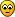Hmm... what's the abstract tag for...Plus, I used Deque because of this.But heh, you're actually using more classes for the same thing. Is that what you call bloated?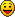Oh, and if I go on with this, I'll give Linoleum a shot. It should be easy to work with integers. # quoteVacuus 11 years ago "E_net4" said:"Amarth" said:Bah. That's way too procedural. While, break, continue, if-then-else... And you're using magic numbers. Also, why use Deque and not just Stack?I read that and went WAT. Then I looked at that code and admitted that was even more Object Oriented than my version.Hmm... what's the abstract tag for...Plus, I used Deque because of this.But heh, you're actually using more classes for the same thing. Is that what you call bloated?Oh, and if I go on with this, I'll give Linoleum a shot. It should be easy to work with integers.Java, by nature, is bloated. Forcing OOP means you're constantly looking for workarounds when you're doing something a little different that doesn't normally need to be an object. Best to stick with what the language does best even if it means implementing the algorithm in a non-standard way with a bit of "bloat" rather than rely on statics and whatnot. # quoteNarvius 11 years ago Wow. Interesting insight into architectureI think I now know the reason why I prefer Ruby for projecteuler.net. # quoteE_net4 11 years ago Project Euler... remind me of finishing my Linoleum library for calculating integers of more than 1 unit.So far, I used my CASIO calculator, Linoleum and Java to solve problems.With the 4-byte limitation, however, I can't do much. I even had to use Java's BigInteger. :/ # quoteMageKing17 11 years ago "Amarth" said:Pythonic solution:`def rpn(string): stack, tokens = [], string.strip().split(" ") for token in tokens: if not token.isdigit(): token = eval("%s%s%s" % (stack[-2],token,stack[-1]) stack = stack[:-2] stack.append(int(token)) return stackstring = raw_input("Your command? ")print rpn(string)`Some slight differences from your code, in particular I first split the string into tokens instead of parsing it in a while-loop, and I don't push on the stack for no reasons :p.Shouldn't it return stack[-1]? I mean, it just seems logical that "4 3 + 0" would result in 0, not 7, pointless though it may be.Also, you should stop writing 2.x code and get with the 3.x program.Here's a slight (untested) update:`def rpn(string): stack, tokens = [], string.strip().split(" ") for token in tokens: if not token.isdigit(): token = eval("{} {} {}".format(stack[-2], token, stack[-1]) stack = stack[:-2] stack.append(int(token)) return stack[-1]string = input("Your command? ")print rpn(string)` # quoteAmarth 11 years ago "MageKing17" said:Shouldn't it return stack[-1]? I mean, it just seems logical that "4 3 + 0" would result in 0, not 7, pointless though it may be.That's not a valid RPN expression in my eyes. Garbage in, garbage out. # quoteE_net4 11 years ago Yeah, I'm working on the Linoleum version for the library. What makes things more complicated are the switch cases, which must be done in low-level. And no, it's not OO.Edit: Oh well. Looks like I haven't managed to fix the code, but it should at least show a possible solution. I used the actual system stack to store the elements, all integers.As a property of Linoleum, you can delete all new lines and spaces. The program will still compile successfully.`( * Reverse Polish Notation library * Made by E_net4 * * Example of use: * rpn . run : [p my command]; * * where [p my command] points to a string of the command. The string should be in UCS-4.)"libraries" data/string/strlen;( data/heap/dynamic/mgrab; ) "directors" unit = 32; namespace = rpn;"constants" char Space = 20h; char Add = 2Bh; char Sub = 2Dh; char Mul = 2Ah; char Slash = 2Fh; char Zero = 30h; char Nine = 39h; status init = 0; status value = 1;"variables"( default command ) null command = 0;( pointer to command ) p command = null command;"workspace" w var; s len; "preambles" leave ( preambles );"run": [p command]; with, [s len] <- string . strlen: [p command]; as, A = 0; ( operand 1 / temp read value / result of single instruction ) B = 0; ( operand 2 / temp value ) C = 0; ( number of elements in stack ) D = [p command]; ( pointer to character in command string ) E = 0; ( supposed result ) [wvar] = status init; ( Current status of reading command... I ran out of registers, ok? ) [s len]; ( length in units of command string ) "run loop" with, B = [D]; (CHECK FOR NUMBER) ? B < char Zero > char type is not number; ? B > char Nine > char type is not number; "char type is number" A * 10; B - char zero; (we now have value of char) A + B; (put value into our number) [wvar] = status value; (we're reading a value for sure) > continue run loop; "char type is not number" ( CHECK FOR SPACE ) ? B ! char Space > char type is not space; ( We found a space character. If status is init, then do nothing. If status is value, then push the value, and set status to init. ) ? [wvar] = status init > continue run loop; --> A; (Push value to stack) + C; (Update nr. of elements) A = 0; [wvar] = status init; > continue run loop; "char type is not space" [wvar] = status init; (CHECK ADDITION) ? B ! char Add > char type is not addition; A = 0; B = 0; ? C = 0 > no op1 to add; <-- B; (Pop op 2) - C; "no op1 to add" ( or done taking op1 ) ? C = 0 > no op2 to add; <-- A; (Pop op 1) - C; "no op2 to add" ( or done taking op2 ) A + B; (Perform operation) --> A; + C; > continue run loop; "char type is not addition" (CHECK SUBTRACTION) ? B ! char Sub > char type is not subtraction; A = 0; B = 0; ? C = 0 > no op1 to subtract; <-- B; (Pop op 2) - C; "no op1 to subtract" ( or done taking op1 ) ? C = 0 > no op2 to subtract; <-- A; (Pop op 1) - C; "no op2 to subtract" ( or done taking op2 ) A - B; (Perform operation) --> A; + C; > continue run loop; "char type is not subtraction" (CHECK MULTIPLICATION) ? B ! char Mul > char type is not multiplication; A = 0; B = 0; ? C = 0 > no op1 to multiply; <-- B; (Pop op 2) - C; "no op1 to multiply" ( or done taking op1 ) ? C = 0 > no op2 to multiply; <-- A; (Pop op 1) - C; "no op2 to multiply" ( or done taking op2 ) A * B; (Perform operation) --> A; + C; > continue run loop; "char type is not multiplication" (CHECK DIVISION) ? B ! char Slash > char type is not dvision; ( D - Vision : for when division is not possible) A = 0; B = 0; ? C = 0 > no op1 to dvide; <-- B; (Pop op 2) - C; "no op1 to dvide" ( or done taking op1 ) ? C = 0 > no op2 to dvide; <-- A; (Pop op 1) - C; "no op2 to dvide" ( or done taking op2 ) A / B; (Perform operation) --> A; + C; > continue run loop; "char type is not dvision" ( And we're done checking the character ) "continue run loop" + D; ( next character ) ? D < [s len] > run loop; ? C = 0 > zero result; <-- E; ( Result is here! ) - C; "zero result" ( There. E holds the result. Now we empty our part of the stack..."empty stack" ? C = 0 > stack is empty; <-- A; (We have to pop it to somewhere...- C; > empty stack; "stack is empty" ( And we're done )return [register] E;` # quoteAmarth 11 years ago Perl!`use Math::RPN;print "Your command? ";\$_ = <>;chomp;@expr = split(' ');\$value = rpn(@expr);print \$value . "\n";`It's probably possible to do it shorter. It's been a long time ago since I abused Perl. # quoteNarvius 11 years ago Oh, there's a built-in RPN module in Perl?What for? # quoteE_net4 11 years ago Heh, that's not fair.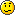By the way, have you noticed something wrong with the Lino code? It should be easy to understand.# quoteNarvius 11 years ago Uh, whateverAlso, infinite cookies for the first brainfuck implementation. xD # quoteMageKing17 11 years ago "Narvius" said:brainfuck implementationHell fuck no. # quoteE_net4 11 years ago Infinite cheezburgers for LOLCODE implementation. # quoteVacuus 11 years ago Y'know I actually did do something very similar in C++ -ages- ago. I might modify that and post it up for the hell of it. Or just rewrite it I guess. # quoteVacuus 11 years ago Here ye C++ implementation.Not perfect and I didn't bother with a lot of error checking but it works, right??`#include #include #include #include #include #include #include template class Operator{ public: virtual valType operator() (valType, valType) = 0;};templateclass Add : public Operator{ valType operator() (valType first, valType second) { return first + second; }};templateclass Subtract : public Operator{ valType operator() (valType first, valType second) { return second - first; }};templateclass Multiply : public Operator{ valType operator() (valType first, valType second) { return first * second; }};templateclass Divide : public Operator{ valType operator() (valType first, valType second) { return second / first; }};template class RPNHandler{ private: // This is a bit of hackery, current C++ implementation doesn't support string literals as non-type template parameters // (Infact it doesn't support any sort of literal, templated paramaters have to be linked globally to one degree or another). // I would have prefered to use template specialisations as it would mean we could bypass this level of indirection // and automatically handle operators, but apparently that'll have to wait until the new standard. // This just provides a map for operator functors through the Operator abstract base class. std::map*> operatorMap; std::stack opStack; public: RPNHandler() { //You can add your own operators as well if need be, they just can't contain any whitespace (fixable but meh) operatorMap["+"] = new Add; operatorMap["-"] = new Subtract; operatorMap["*"] = new Multiply; operatorMap["/"] = new Divide; } void operator()(std::string token) { std::istringstream stream(token); valType digit; if (stream>>digit) { //Token is a number opStack.push(digit); } else { //Assume it's an operator if (operatorMap.find(token) == operatorMap.end() || opStack.size() < 2) { throw std::runtime_error("Invalid RPN Expression"); } valType first = opStack.top(); opStack.pop(); valType second = opStack.top(); opStack.pop(); opStack.push( (*operatorMap[token](first, second)); } } valType result() { if (opStack.size() != 1) { throw std::runtime_error("Invalid RPN Expression"); } return opStack.top(); }};int main(int argc, char** argv){ std::cout<<"Your command? "; std::string input; std::getline(std::cin, input); //Tokenise the input (split on whitespace) std::stringstream stream(input); std::istream_iterator tokens_begin(stream); std::istream_iterator tokens_end; RPNHandler rpn; //Probably an STL algorithm for this... for (std::istream_iterator it = tokens_begin; it != tokens_end; ++it) { rpn(*it); } std::cout<<"\n"<http://pastebin.com/zftjbdCtNext up? Lua, just to show Amarth it's actually worth learningEdit: Oops, just noticed that the result function in RPNHandler has a return type of int and not valType. Give me a break, it's 4am sheesh. # quoteVenom31 11 years ago "Vacuus" said:class RPNHandler{ private:...Usually the class starts by private members as default, you don't have to specify it. Except for those who do not understand C++ :sic: # quoteE_net4 11 years ago We know that, but it's there for the sake of readability. What kind of intervention was that?# quoteAmarth 11 years ago I like it... Get the Lua on! # quoteVacuus 11 years ago "Venom31" said:"Vacuus" said:class RPNHandler{ private:...Usually the class starts by private members as default, you don't have to specify it. Except for those who do not understand C++ :sic:Yep, private is the default access specifier but people that use java and other wouldn't know what. Like E_net4 said it's just for readability. I also missed a couple of (trivial) things such as adding operators dynamically through a function and so on; it was late, I was tired and I think you get the idea anywayHere's an example using lua, again I missed a little bit of error checking but it's fairly clean, right?`function string:split(splitter) local splitter, fields = splitter or " ", {} local pattern = string.format("([^%s]+)", splitter) self:gsub(pattern, function(c) fields[#fields+1] = c end) return fieldsendfunction rpnEquation() local self = {} --Mimics an object; lua tables are very powerful local stack = {} function self.proc(token) if tonumber(token) then table.insert(stack, 1, tonumber(token)) else --Assume it's an operator. assert( #stack >= 2, "Invalid RPN Equation") first, second = stack, stack --Better ways to do this but I'm lazy table.remove(stack, 1) table.remove(stack, 1) table.insert(stack, 1, assert(loadstring("return " .. second .. token .. first) )() ) end end function self.result() return stack end return selfendio.write("Your command? ")input = io.read("*line")rpn = rpnEquation()for _,token in ipairs(input:split(" ")) do rpn.proc(token) endprint(rpn.result())print("Press enter to quit... ")io.read()`Pastebin: http://pastebin.org/323026Okay, so it's not the best introduction to lua in the world, but even so. # quoteE_net4 11 years ago Know what I just thought of? An Assembly implementation.# quoteNarvius 11 years ago Did rewrite into more general thingy because of borednessdom.`class Array def but_first return self[1, length] endendclass RPNStack < Array def initialize(type = Numeric) @type = type end def execute stack = [] for item in self if item.is_a? @type then stack.push(item) elsif item.is_a? Symbol arity = stack[-1].method(item).arity items = stack.pop(arity < 0 ? 2 : arity + 1) stack.push(items.send(item, *(items.but_first))) else raise("Incompatible object pushed on RPNStack.") end end return stack endend`I can now create RPN stacks that can handle any declared Ruby function, or work on strings instead of numbers and so on.Since there aren't many string functions that take other strings as argument, pretty useless... but was interesting to write, nonetheless.Also, this time only contains one instance of Ruby Magic.`rpn = RPNStack.newfor token in gets.strip.split(" ") if ("0".."9") === token or token == "-" if token.include? "." rpn.push(token.to_f) else rpn.push(token.to_i) end else rpn.push(token.to_sym) endendputs rpn.execute`And some test coed.Parses amusing things like "5 4 1 modulo 4 7 lcm gcd +". # quote
Forum » Reverse Polish Notation!Your email: Your name: